# Shuffling a table

This page is about an algorithm which can shuffle a table into a random order, called the Fisher-Yates shuffle.

Let's say you have a group of things, like these shapes on the right.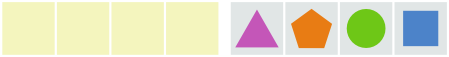And you want to put them in a random order into the area on the left.

What you could do is pick a random shape and place it in the area on the left.

Let's say we picked the circle. Let's move it to the other side.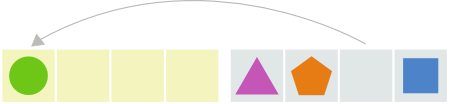And now we can just repeat. Pick a random shape, and move it to the other side.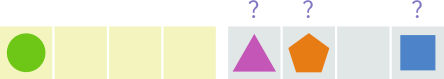Let's pick the pentagon.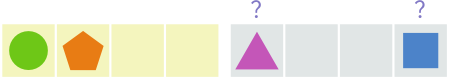And now the square.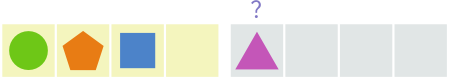And now we don't have a choice, let's move the remaining triangle over too.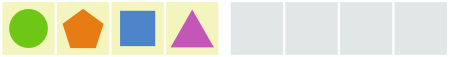And that's it! Shuffled.

## Now the same thing, except without all the blank spaces.

Let's do this again. We'll pick the same shapes as last time, so first up is the circle.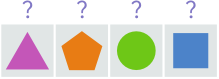And then, let's move it over to the left, and shift everything across.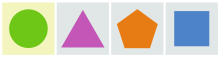So now we pick another random shape from the area on the right. I'll put a red flag to show where the area on the right starts.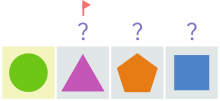Let's pick the pentagon.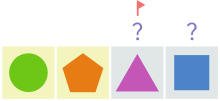And now the square.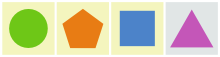And hey, turns out the last shape is already there.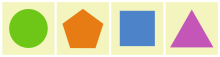So, that example was the same thing as the one before. If that wasn't clear, here's both of them side by side: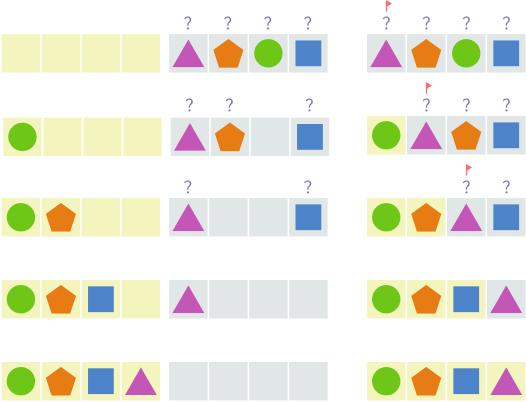Okay, now this time it'll be ever-so-slightly different. Instead of shifting across the shapes on the right when one of them is placed in the area on the left, we'll swap them. You'll see what I mean.

So let's do this again, and pick a random shape, which will be the circle.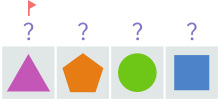Now let's move it to the area on the left, swapping it with the triangle under the flag.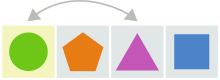This is basically the same as the other examples after one move, except the order of the shapes in the area on the right is different. The order of the shapes on the right doesn't matter though, since we'll be picking one from there randomly anyway.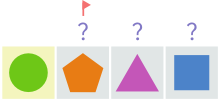Now let's pick another random shape from the area on the right, the pentagon, and swap it with whatever is under the flag, which is itself. Not a problem.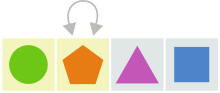Now let's pick another random shape from the area on the right, the square, and swap it with the triangle under the flag.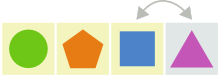And there's only one left, and it's already in the only place it can be, so we're done.So how could this algorithm be written in English? How about...

• Pick a random element between the flag and the last element.
• Swap it with the element at the flag.
• Move the flag along
• Repeat the last three steps, stopping when the flag is at last element.

Let me conveniently rephrase that into something more like the Lua code...

Start a loop, with a flag starting at the first element and moving along every time, and when the flag is at one-before-the-last-element this will be the final iteration, and do the following things inside the loop:

• Pick a random element between the flag and the last element
• Swap it with the element at the flag

Now here's that in Lua:

``````function shuffle(t)
for i = 1, #t - 1 do
local r = math.random(i, #t)
t[i], t[r] = t[r], t[i]
end
end
``````

So, let's see how this works with a run through.

Let's give this function our table of shapes...

``````function shuffle({'triangle', 'pentagon', 'circle', 'square'})
for i = 1, 3 do
local r = math.random(i, 4)
t[i], t[r] = t[r], t[i]
end
end
``````

i is the flag! It starts at 1, and loops through 1-less-than-the-number-of-elements times, which is 3 in this case.

Let's see what happens on the first iteration of the loop...

``````function shuffle({'circle', 'pentagon', 'triangle', 'square'})
for i = 1, 3 do                  -- i is now 1
local r = math.random(1, 4)  -- Let's say r is now 3
t, t = t, t      -- 'triangle' swaps with 'circle'
end
end
``````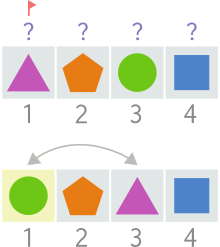And the second...

``````function shuffle({'circle', 'pentagon', 'triangle', 'square'})
for i = 1, 3 do                  -- i is now 2
local r = math.random(2, 4)  -- Let's say r is now 2
t, t = t, t      -- 'pentagon' swaps with itself
end
end
``````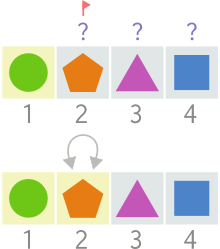And the third and final iteration...

``````function shuffle({'circle', 'pentagon', 'square', 'triangle'})
for i = 1, 3 do                  -- i is now 3
local r = math.random(3, 4)  -- Let's say r is now 4
t, t = t, t      -- 'triangle' swaps with 'square'
end
end
``````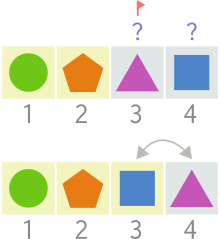And the table has been shuffled!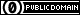To the extent possible under law, the person who associated CC0 with this work has waived all copyright and related or neighboring rights to this work.